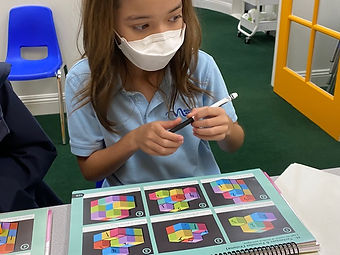## Ms. Dawn

### Target 1​

###### Lesson Type:

Continuation

Measurment

:

Techniques and Formulas

Explore the concept of volume using blocks.

###### 1:

Understand that volume is the measure of the amount of space inside a solid figure.

###### 2:

Understand that cubes can be counted to determine the volume.

###### 3:

Utilize different strategies for counting volume cubes.

4th

###### Vocabulary:

Volume, Cubes, Space, Solid figure, Layers, Sections, Combine

Activities:

1. Revisited the definition of what volume is and the formula.

2. Students were given a variety of pictures that showed cubes arranged differently. They found the volume of the shape by using strategies to breaking down the shape into parts. They used the idea of breaking the shapes into layers, sections, etc.

3. Students wrote the formula down on their whiteboards and showed how they computed each part of the arranged cubes. They split the irregular shape by using a pen to trace where they separated the layers or sections. Then, they solved each part and combined it together to find the total volume.

4. Students used blocks to build the shape to help them see it visually and split it be separting it by how they would solve each part.### Home Exploration

###### Guiding Questions:## Absent Students:

### Target 2

:

###### 1:

Understand equivalent measurements.

###### 2:

Understand how to convert from one unit to another.

###### Vocabulary:

Equivalent, Covert, Unit, Measurement, Conversion chart, Length, Mass, Weight, Capacity, Time, Metric, Customary

Activities:

1, Discussed the different types of measurment and how it is used in real life.

2. Discussed what it means to convert one measurment to another. Started with simple things like, 2ft.= how many inches. Students answered a few problems like these their whiteboard by using the conversion chart.

3. After completing a few problems, students played a game called "Rock, Paper, Scissor," Each students started at a "Start" line by placing their cube color on it. They challenged rock, paper, scissor. The student who won had to move their cube up and solve the measurement problem. They needed to convert one unit to another. The first person to the finish line won.

4. Next activity we started was to answer measurement problems and each one related to a feature for a picture they will end up creating.### Home Exploration

###### Guiding Questions:### Target 3

:

###### Vocabulary:

Activities:### Home Exploration